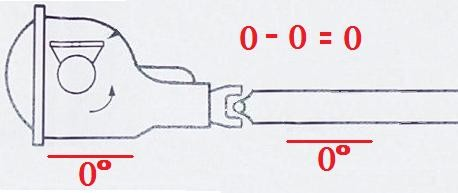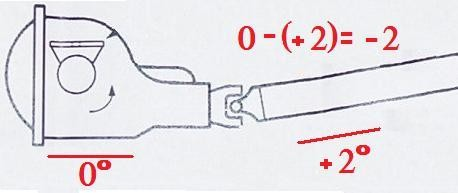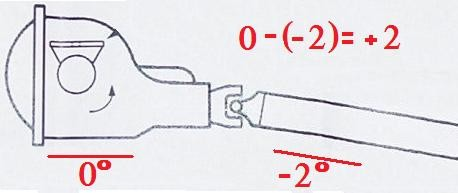# Measuring Pinion Angle by JonCR96Z

By diyauto
( 3 )Measuring Pinion Angle

Compliments of JonCR96Z @ https://ls1tech.com

8-21-2008

Ok, after arguing about it in one thread and reading plenty of others about finding pinion angle with varying explanations, this needs to be cleared up and made a little bit easier to understand.

Some of the confusion comes from one person measuring the pinion angle from the drivers side of the car and others measuring from the passenger side and the rest of the confusion is from the adding and subtracting of the rearend and driveshaft angles.

For this thread I want to try to keep the discussion as if you were looking at the measurements from the passenger side, like the illustrations below and the guy in the BMR video (which I'm gonna bring up in a minute). If you measure pinion angle from the drivers side then the measurements and the formulas will be inverted. So assuming we're looking at it from the passenger side, then throughout this post I'm going to keep the rearend angles on the left and the driveshaft angles on the right.
Example: A rearend angle of (-3) and a driveshaft angle of (+1) will be shown as (-3)/(+1).

1. Getting the car ready to measure pinion angle.

In order to correctly measure pinion angle your car needs to be fairly level from front to back with the weight of the car supported by all four tires and nothing else. Just as if it parked in your driveway or sitting in the staging lanes. The reason for this is that if the car is supported by jack stands, the rearend would hang lower than normal and change the angle of the driveshaft and torque arm, since they are mounted to either the body of the car or the transmission (which is also mounted to the body), which would cause your measurements to be horribly wrong at best.

Using a magnetic angle finder you can stick it to the bottom of the torque arm mounting bracket. This will allow you to measure the angle of the rearend. To find the driveshaft angle you need to put it on the driveshaft, which for us aluminum driveshaft guys means that you'll have to hold it there. So just find a clean spot on the driveshaft and either stick or hold it there until it stops moving and record that number. If you do have an aluminum driveshaft and are having to hold it in place, I recommend repeating the process a few times, just to double check that you are getting the same number. Having the angle finder tilted just slightly from side to side could affect your measurement.

2. Zero Degrees.

First off, lets start with what zero degrees of pinion angle is. This is when the angle of the rearend and the angle of the driveshaft are the same. Example: (-3)/(-3) or (+4)/(+4) or (0)/(0) or any measurement that has the mounting bracket of the torque arm and the driveshaft parallel. Easy enough, right? Now understanding what zero degrees is, lets move on to the negative angles.3. Negative Pinion Angles.

For a negative pinion angle, the rearend has to be angle downward (from back to front) from the measurement of zero degrees. For this post we will use negative two degrees, as it is the most common and most recommend starting point. The formula to use is for finding pinion angle is: (rearend angle)-(driveshaft angle)=(pinion angle). And remember that subtracting a negative is the same as adding a positive. Examples: (+2)-(-3)=(+5) , (-2)-(-3)=(+1) , (-2)-(+3)=(-5) , (+2)-(+3)=(-1).

While setting a negative two degree pinion angle, you only want a two degree spread from rearend angle to driveshaft angle. Some examples are: (-2)/(0) or (-4)/(-2) or (-1)/(+1) or (0)/(+2). As long as the measurements are two degrees apart with the rear tilted downward from back to front, in relation to the driveshaft.4. Positive Pinion Angles.

This is the opposite of the above, where the rearend tilts upward form back to front in relation to the driveshaft. For this I'm just going to list some examples and give their overall pinion angle. (0)/(-2)=positive two degrees, (+5)/(+1)= positive four degrees, (-2)/(-4)= positive two degrees, (+3)/(-2)=positive five degrees.5. The BMR video.

BMR uses a different method of measuring the angles. As explained below in post #13, by Allen Miller https://ls1tech.com/forums/11216876-post13.html